# Effects of Change of External Conditions in Dynamic Equilibrium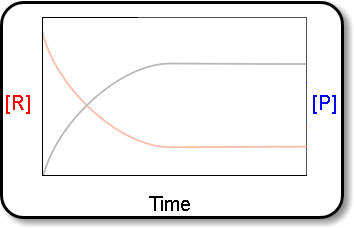↪ A French chemist Le Chatelier studied the effect of pressure, temperature and concentration on a large number of chemical equilibria and consequently he introduced a principle of mobile equilibrium which is commonly called as Le Chatelier's principle.

Statement:

↪ If a system at a state of dynamic equilibrium is subjected to a constraint (stress), the equilibrium shifts in such a direction so as to reduce the effect of applied constraint.

↪ The principle is applicable both for chemical and physical equilibria.

Applications in Chemical Equilibrium

↪ ﻿Pressure, temperature, concentration and catalyst are the constraints which can be applied to the chemical equilibria and the effects they produce are studied as follows:

⁕ Effect of Pressure

↪ The effect of increase of pressure in a given equilibrium is reduced by shifting the equilibrium along the direction where the total volume is decreased.
↪ The decrease of pressure shifts the equilibrium towards the direction where volume is increased.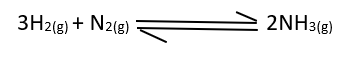Total volume of gaseous reaction = 1+3 = 4
Total volume of gaseous reaction = 2

↪ When the external pressure is increased in this equilibrium, the equilibrium shifts to forward direction because it involves the decrease of volume.
↪ Similarly, the decrease of pressure shifts the equilibrium to the backward direction because the path is accompanied by increase of volume.

⁕ Effect of Temperature

↪ A reversible reaction if it is exothermic in the forward direction must be endothermic backward and vice versa.
↪ The effect of increase of temperature in the equilibrium is relieved by shifting the equilibrium towards the endothermic path where heat is absorbed.
↪ On the other hand the equilibrium shifts towards the exothermic  path when temperature is decreased in the equilibrium.

Consider some examples: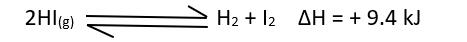↪ The thermo chemical equation tells that the decomposition of hydrogen iodide is endothermic and the equilibrium shifts to the forward direction i.e., the decomposition of HI will be more when the temperature of reaction is increased.
↪ The formation of HI is favored when the temperature in the equilibrium is decreased because this path is exothermic in nature.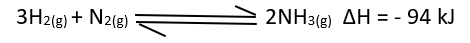↪ The formation of ammonia is exothermic process whilst the decomposition of ammonia is endothermic.
↪ According to the principle, low temperature shifts the equilibrium to the forward path and formation of NH3 is favored.
↪ High temperature shifts the equilibrium to the backward path and the decomposition of NH3 is favored.

Effect of Concentration

↪ If the concentration of one of the components of equilibrium is increased by extra addition, the system tends to relieve the stress of concentration by shifting the equilibrium along that direction where its concentration decreases.
↪ Similarly, decrease of concentration of the components from the equilibrium mixture shifts the equilibrium along as direction so that more of its amount undergoes formation.

For example, decomposition of﻿ CaCO3 in a close vessel attains equilibrium as: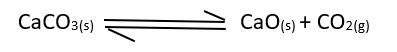↪ If CO2 gas present in the equilibrium is removed the reaction forwards so as to compensate the loss of CO2 for the equilibrium.
↪ If more CO2 is injected in the close container the concentration of CO2 will be more and equilibrium shift to the backward direction and the stress of concentration in product side is relieved.

⁕ Effect of Catalyst

↪ ﻿Addition of catalyst increases the rates of both forward and backward oaths of a reversible reaction and it makes the reaction to attain equilibrium rapidly without changing the equilibrium concentration of reactants and products.
↪ A catalyst alter the rates of chemical reaction without changing the position of dynamic chemical equilibrium and equilibrium constant.

Application to Physical Equilibrium

↪ Melting, freezing, evaporation, condensation, ionization, solubility, etc. are processes involving physical equilibria.
↪ Le Chatelier principle can also be applied here to predict the position of equilibrium affected by temperature and pressure.

⁕ Melting and Freezing

↪ Melting and freezing are the processes reverses to each other.
There exists an equilibrium between solid and liquid at melting point of solid and freezing point of liquid.
↪ The melting point and freezing point are identical temperature for a given pure substance,
Effects of temperature and pressure on the melting and freezing of a substance are discussed in brief as follows:

⁕ Effects of temperature

↪ The equilibrium between a solid and liquid of a substance is represented as:⁕ Effects of Pressure

↪ A solid having volume greater than its liquid can melt even below its normal melting point by increase of pressure i.e., pressure lowers the melting point of such solid.
↪ On the other hand pressure rises the melting point of those solids having their volume less than their melts e.g., paraffin.

⁕ Evaporation and Condensation

↪ ﻿Evaporation of a liquid and condensation of a vapor are the processes reverse to each other.
↪ These processes attain a state of equilibrium in a close system as:↪ Evaporation is a process accompanied by the increase of volume and condensation involves a decrease of volume.
↪ Hence, increase of pressure facilitates the process of evaporation of a liquid.

↪ Further, evaporation involves the absorption of heat and condensation involves evolution fo heat.
↪ Hence increase of temperature favors evaporation and decrease of temperature favors condensation.